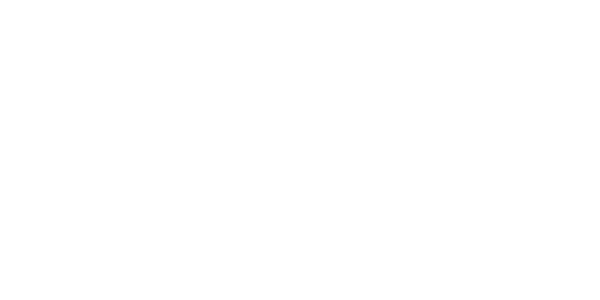LATEST >>

Welcome Here And Thanks For Visiting. Like Us On Facebook...

# Top 5 Awesome JavaScript Snippets For Common TasksIn this article we’re going to cover the following popular script snippets!Web developers often use JavaScript for common tasks on their websites. In this tutorial we’ll show you the top 10 JavaScript snippets you can use on your webpages by just cutting and pasting!

1. Date display
2. Calendar
3. Copy Selected Text
4. Break out of Frames

## 1.) Date Display:

You can use this snippet to display the current date in this format: Friday, June 11, 2010. Just paste the following code where you want the date to show up on your page:

```<script type="text/javascript">
var now = new Date();
var days = new Array('Sunday','Monday','Tuesday','Wednesday','Thursday','Friday','Saturday');
var months = new Array('January','February','March','April','May','June','July','August','September','October','November','December');
var date = ((now.getDate()<10) ? "0" : "")+ now.getDate();
function fourdigits(number) {
return (number < 1000) ? number + 1900 : number;
}
today =  days[now.getDay()] + ", " +
months[now.getMonth()] + " " +
date + ", " +
(fourdigits(now.getYear())) ;
document.write(today);
</script>```

## 2.) Calendar:

This is a plain and simple monthly calendar. It’s formatted with styles sheets so the appearance can easily be changed. In the head of your document, add the following style sheet and JavaScript code:

```<style type="text/css">
span.label {color:black;width:30;height:16;text-align:center;margin-top:0;background:#ffF;font:bold 13px Arial}
span.c1 {cursor:hand;color:black;width:30;height:16;text-align:center;margin-top:0;background:#ffF;font:bold 13px Arial}
span.c2 {cursor:hand;color:red;width:30;height:16;text-align:center;margin-top:0;background:#ffF;font:bold 13px Arial}
span.c3 {cursor:hand;color:#b0b0b0;width:30;height:16;text-align:center;margin-top:0;background:#ffF;font:bold 12px Arial}
</style>
<script type="text/javascript">
function maxDays(mm, yyyy){
var mDay;
if((mm == 3) || (mm == 5) || (mm == 8) || (mm == 10)){
mDay = 30;
}
else{
mDay = 31
if(mm == 1){
if (yyyy/4 - parseInt(yyyy/4) != 0){
mDay = 28
}
else{
mDay = 29
}
}
}
return mDay;
}
function changeBg(id){
if (eval(id).style.backgroundColor != "yellow"){
eval(id).style.backgroundColor = "yellow"
}
else{
eval(id).style.backgroundColor = "#ffffff"
}
}
function writeCalendar(){
var now = new Date
var dd = now.getDate()
var mm = now.getMonth()
var dow = now.getDay()
var yyyy = now.getFullYear()
var arrM = new Array("January","February","March","April","May","June","July","August","September","October","November","December")
var arrY = new Array()
for (ii=0;ii<=4;ii++){
arrY[ii] = yyyy - 2 + ii
}
var arrD = new Array("Sun","Mon","Tue","Wed","Thu","Fri","Sat")
var text = ""
text = "<form name=calForm>"
text += "<table border=1>"
text += "<tr><td>"
text += "<table width=100%><tr>"
text += "<td align=left>"
text += "<select name=selMonth onChange='changeCal()'>"
for (ii=0;ii<=11;ii++){
if (ii==mm){
text += "<option value= " + ii + " Selected>" + arrM[ii] + "</option>"
}
else{
text += "<option value= " + ii + ">" + arrM[ii] + "</option>"
}
}
text += "</select>"
text += "</td>"
text += "<td align=right>"
text += "<select name=selYear onChange='changeCal()'>"
for (ii=0;ii<=4;ii++){
if (ii==2){
text += "<option value= " + arrY[ii] + " Selected>" + arrY[ii] + "</option>"
}
else{
text += "<option value= " + arrY[ii] + ">" + arrY[ii] + "</option>"
}
}
text += "</select>"
text += "</td>"
text += "</tr></table>"
text += "</td></tr>"
text += "<tr><td>"
text += "<table border=1>"
text += "<tr>"
for (ii=0;ii<=6;ii++){
text += "<td align=center><span class=label>" + arrD[ii] + "</span></td>"
}
text += "</tr>"
aa = 0
for (kk=0;kk<=5;kk++){
text += "<tr>"
for (ii=0;ii<=6;ii++){
text += "<td align=center><span id=sp" + aa + " onClick='changeBg(this.id)'>1</span></td>"
aa += 1
}
text += "</tr>"
}
text += "</table>"
text += "</td></tr>"
text += "</table>"
text += "</form>"
document.write(text)
changeCal()
}
function changeCal(){
var now = new Date
var dd = now.getDate()
var mm = now.getMonth()
var dow = now.getDay()
var yyyy = now.getFullYear()
var currM = parseInt(document.calForm.selMonth.value)
var prevM
if (currM!=0){
prevM = currM - 1
}
else{
prevM = 11
}
var currY = parseInt(document.calForm.selYear.value)
var mmyyyy = new Date()
mmyyyy.setFullYear(currY)
mmyyyy.setMonth(currM)
mmyyyy.setDate(1)
var day1 = mmyyyy.getDay()
if (day1 == 0){
day1 = 7
}
var arrN = new Array(41)
var aa
for (ii=0;ii<day1;ii++){
arrN[ii] = maxDays((prevM),currY) - day1 + ii + 1
}
aa = 1
for (ii=day1;ii<=day1+maxDays(currM,currY)-1;ii++){
arrN[ii] = aa
aa += 1
}
aa = 1
for (ii=day1+maxDays(currM,currY);ii<=41;ii++){
arrN[ii] = aa
aa += 1
}
for (ii=0;ii<=41;ii++){
eval("sp"+ii).style.backgroundColor = "#FFFFFF"
}
var dCount = 0
for (ii=0;ii<=41;ii++){
if (((ii<7)&&(arrN[ii]>20))||((ii>27)&&(arrN[ii]<20))){
eval("sp"+ii).innerHTML = arrN[ii]
eval("sp"+ii).className = "c3"
}
else{
eval("sp"+ii).innerHTML = arrN[ii]
if ((dCount==0)||(dCount==6)){
eval("sp"+ii).className = "c2"
}
else{
eval("sp"+ii).className = "c1"
}
if ((arrN[ii]==dd)&&(mm==currM)&&(yyyy==currY)){
eval("sp"+ii).style.backgroundColor="#90EE90"
}
}
dCount += 1
if (dCount>6){
dCount=0
}
}
}
</script>```

Then, in the body of your document, add the following where you want the calendar to show up:

`<script type="text/javascript">writeCalendar()</script>`

## 3.) Copy Selected Text:

Often you may have some information on your page that your visitors might want to copy. You can make it easier for them by providing a mechanism that allows them to simply click a button to do so. First you need to paste this code into the head of your web page:

```<script type="text/javascript">
function copyit(theField) {
var selectedText = document.selection;
if (selectedText.type == 'Text') {
var newRange = selectedText.createRange();
theField.focus();
theField.value = newRange.text;
} else {
alert('select a text in the page and then press this button');
}
}
</script>```

And in the body of your web page, add the following where you want the text to appear:

```<form name="it">
<div align="center">
<input onclick="copyit(this.form.select1)" type="button" value="Press to copy the highlighted text" name="btnCopy">
<p>
<textarea name="select1" rows="4" cols="45"></textarea>
</div>
</form>```

## 4.) Break Out Of Frames:

To stop your page being framed by someone else, which isn’t a common practice any longer thankfully, put this event handler into the body tag of your document:

`<body onLoad="if (self != top) top.location = self.location">`

Often you may want to let the visitor know that you are aware of their IP address. You can do so using a few lines of code, as shown here. Place the code where you want the IP address to appear:

```<script type="text/javascript">